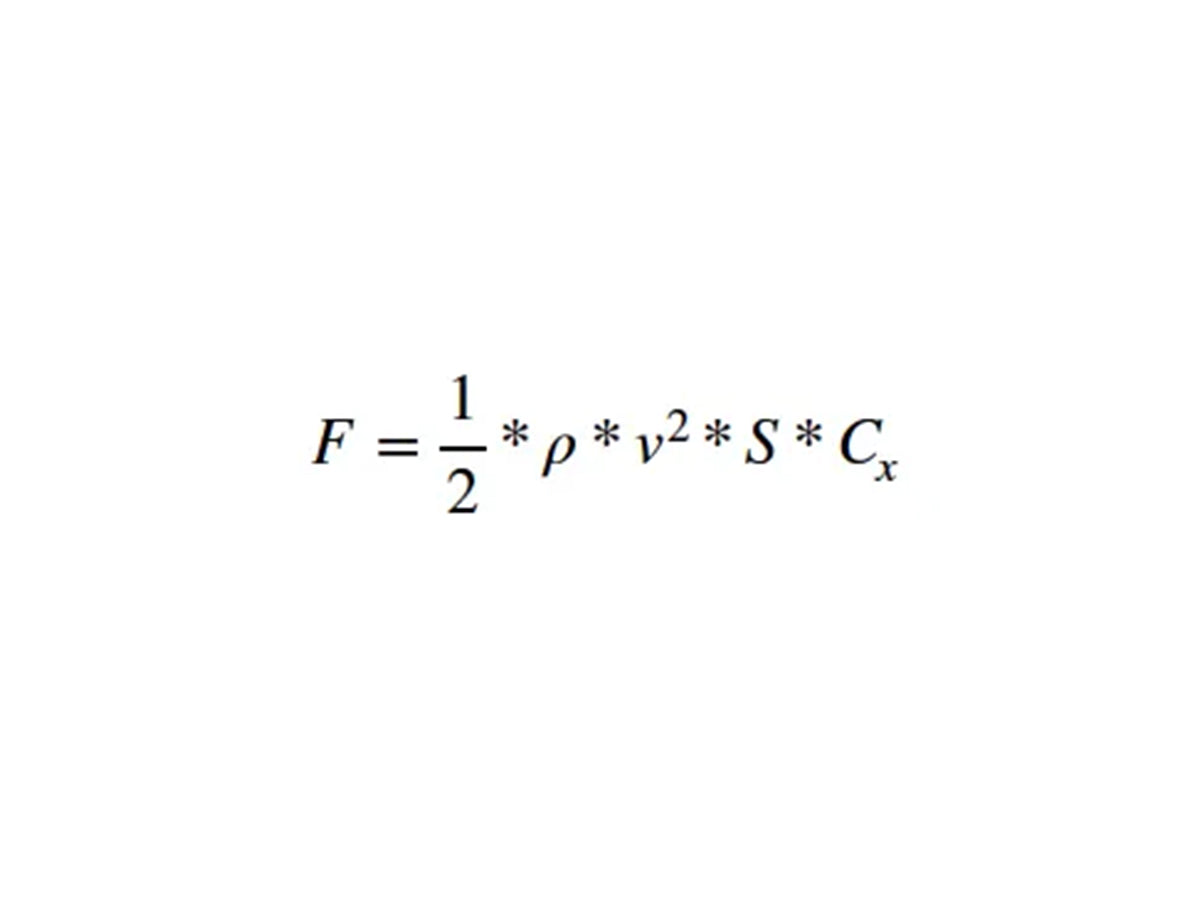# Calculating wind resistance

At Shapeheart, we like to calculate.

So we're going to put them down to explain how our patented magnet system is able to lift more than 8kg and still resist wind speed.

And we also explain it all to you in video 👉## Formula

In turbulent flow, the force applied by the wind on the sleeve is expressed as shown opposite.

Don't panic, we detail all the parameters below:

- r being the density of the air = 1,292 kg.m-3
- v being the speed in m.s-1
- S the surface of the sleeve = 18 * 10 cm2
- Cx the drag coefficient = 1,1
- F being the force expressed in NewtonFor 8kg = 80N (N for Newton) of force of our magnetic system, the maximum theoretical speed is thus obtained with the formula:.## Max. theoretical speed

If we plot this curve, we get :

in Y : the speed

in X : the magnetizing force in NThis gives for 80 N or 8 kg of force of the magnetic system the speed of 285 km/h (177mph) :

It should be noted that in reality, this speed is probably twice as high:

The smartphone is never set at 90° (vertical) to the wind but rather at 45° of the structure of the motorcycle breaks and slows down the air flow near the handlebars